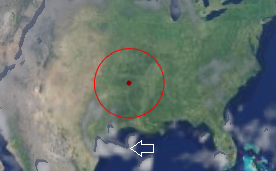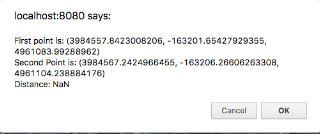# Distance between cartesian points

I have two cartesian points and I want to know the distance between them. I wrote the follow function:

function distance(p1, p2) {

return Math.sqrt(Math.pow((p1.x - p2.x), 2) + Math.pow((p1.y - p2.y), 2));

}

But it is not right.

My application draws a circle with mouse. When the mouse moves on horizontal It’s ok:But on vertical the radius is lesser:where p1 is center and p2 is the mouse position. It’s a cartesian coordinates problem?

Thanks!

Hi Tamy,

The problem is that you are measuring the straight line distance between the two coordinates and not accounting for the curvature of the earth. So while your distance computation is correct, it’s not what you want. What you want to compute is surface distance between the two points. I’m not sure we have a method in Cesium to do this yet (someone please correct me if I’m wrong). We know how, and we have code we can port to JavaScript, we just haven’t done it yet. I can look into adding it in the next few days if that’s okay with you.

Matt

Tamy,

@kring just pointed out to me that you are also missing the z value in your computation, it should be:

function distance(p1, p2) {

return Math.sqrt(Math.pow((p1.x - p2.x), 2) + Math.pow((p1.y - p2.y) + Math.pow((p1.z - p2.z), 2));

}

This is still technically incorrect for large distances, but should get you much closer to what you want until we add actual surface distance calculation for you.

Matt

Matt,

Including z value works perfect for me.

Thanks very much!

Hello,
as Matthew Amato noticed, your formula measures a straight line distance. To take the curvature of the Earth, I suggest you this algorithm (from cartographic points A and B, in radians):

var result;
//arc cos (sinLambdaA sinLambdaB + cos LambdaA cosLambdaB cosDeltaLongitude)*EarthRadius
result=Math.cos(A.getLongitude()-B.getLongitude());
result=result*Math.cos(A.getLatitude())*Math.cos(B.getLatitude());
result=result+Math.sin(A.getLatitude())*Math.sin(B.getLatitude());
result=Math.acos(result);
return result;

Hi.

Is there a measure distance/area control now available?

Or we need to use the below algorithm implementation. If so, what is the accuracy of the same. I need area measurement function also.

Hello the below algorithm is the most precise, see http://en.wikipedia.org/wiki/Haversine_formula .
for Area measurement, I give you another snippet to test (good precision):
/**

Hi All,

I came across this post while looking for an algorithm to calculate the distance between two Cartesian points in Cesium.

However, the function is currently returning ‘NaN’ and not the distance between the two points.

Do you have any idea why it would be doing this?

See code below:

``````function calculateDistance(p1, p2) {
return Math.sqrt(Math.pow((p1.x - p2.y), 2) + Math.pow((p1.y - p2.y) + Math.pow((p1.z - p2.z), 2)));
}
``````
``````
``````

if (clickPositions.length === 2) {

``````var distance = calculateDistance(new Cesium.Cartesian3.fromArray(clickPositions), new Cesium.Cartesian3.fromArray(clickPositions));
console.log("Distance: " + distance);
confirm("First point is: " + clickPositions +
"\nSecond Point is: " + clickPositions +
"\nDistance: " + distance);
``````

} else {
confirm(“You have clicked on more than two points”);
}Thanks,

Chris

I think your calculateDistance() is incorrect, it should be the sqrt() of the added squares of dX, dY and dZ.

Otherwise, try with Cesium.Cartesian3.distance(left, right) : see https://cesiumjs.org/Cesium/Build/Documentation/Cartesian3.html?classFilter=cart#.distance

Regards, WIllem

Hi William,

I agree, the calculateDistance() was not working correctly.

I did however find a solution by adapting a algorithm I found, which uses the cartographic positions instead and takes into account the height value for each point.

I was also going to ask, for the above area algorithm, if the positions array that is passed to Cesium.Ellipsoid.WGS84.cartesianArrayToCartographicArray() is in Radians will the algorithm still work?

I ask because Radians seems to be the default unit for Cartesian3 instances in Cesium.

Cheers,

Chris

Hi Chris,

The stackoverflow solution calculates the distance over the earth’s curved surface, which is very different from the Cartesian3.distance() (straight line between two points).

Cartesian3 is always meters so that will not work with radians. In the algorithm the cartesianArrayToCartographicArray() is meant to convert X,Y,Z to latitude,longitude,height (in radians and meters).